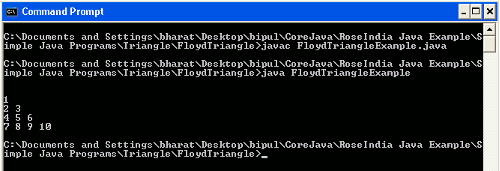# Java Program Floyd's Triangle

In this section you will read about how to write a Java program to print Floyd's triangle.## Java Program Floyd's Triangle

In this section you will read about how to write a Java program to print Floyd's triangle.

This tutorial is about printing a Floyd's triangle in Java. Here you will learn about all the steps for creating the Floyd's triangle in Java.

Before writing a Java program for displaying Floyd's triangle, first we will discuss what is Floyd's triangle.

Floyd triangle is named after a computer scientist Robert Floyd. This triangle is a right angled triangle made up by the array of natural numbers. Rows of this triangle contains the consecutive numbers started from the number '1' in the top left corner. And the sum of n'th row is n(n^2+1)/2.

Floyd's triangle should be look like below :

```1
2 3
4 5 6
7 8 9 10```

Source Code

FloydTriangleExample.java

```public class FloydTriangleExample
{
public static void main(String[] args) throws Exception
{
int k=0;
System.out.println();
for(int i = 0; i <5; i++)
{
for(int j = 0; j <i; j++)
{
k = k + 1;
System.out.print(k);
System.out.print(" ");
}
System.out.println("");
}
}
}```

Output :

When you will execute the above example you will get the output as follows :